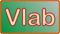onlineoverland:   Overland flow using the diffusion wave method --- One bookReferences Ponce, V. M. 1986. Diffusion wave modeling of catchment dynamics. Journal of Hydraulic Engineering, 112(8), August, 716-727. Ponce, V. M. 1989. Engineering Hydrology: Principles and Practices, Prentice Hall, Englewood Cliffs, New Jersey, 322-325.

 SAMPLE INPUT: [Main Page] [Rationale] [Description]

SI units (metric)

Rainfall (precipitation) depth P :  24

Runoff curve number CN :  100

Rainfall duration tr :  12

Number of points in cumulative rainfall distribution Np :  2

Dimensionless cumulative time for rainfall distribution T* :  0.,1.

Dimensionless cumulative depth for rainfall distribution D* :  0.,1.

Total simulation time Tt :  48

Number of time intervals NΔt :  96

Number of time intervals for printing Np :  1

Fraction used to estimate reference discharge Fr :  [leave blank]

Overland flow area A :  18

Fraction of area in left plane Flp :  0.5

Slope of left plane Slp :  0.001

Manning coefficient for left plane nlp :  0.1

Rating exponent for left plane βlp :  -1

Slope of right plane Srp :  [leave blank]

Manning coefficient for right plane nrp :  [leave blank]

Rating exponent for right plane βrp :  [leave blank]

Length of channel Lch :  400

Slope of channel Sch :  0.01

Manning coefficient of channel nch :  0.015

Bottom width of channel Bch :  2

Design depth of channel ych :  0.6

Side slope of channel z [z H : 1 V] z :  3.

U.S. Customary units

Rainfall (precipitation) depth Pe :  2

Runoff curve number CN :  100

Rainfall duration tr :  12

Number of points in cumulative rainfall distribution Np :  2

Dimensionless cumulative time for rainfall distribution T* :  0.,1.

Dimensionless cumulative depth for rainfall distribution D* :  0.,1.

Total simulation time Tt :  48

Number of time intervals NΔt :  96

Number of time intervals for printing Np :  1

Fraction used to estimate reference discharge Fr :  [leave blank]

Number of books Nb :  1

Overland flow area A :  6

Fraction of area in left plane Flp :  0.5

Slope of left plane Slp :  0.001

Manning coefficient for left plane nlp :  0.1

Rating exponent for left plane βlp :  -1

Slope of right plane Srp :  [leave blank]

Manning coefficient for right plane nrp :  [leave blank]

Rating exponent for right plane βrp :  [leave blank]

Length of channel Lch :  500.

Slope of channel Sch :  0.01

Manning coefficient of channel nch  0.015

Bottom width of channel Bch :  2.

Design depth of channel ych :  0.5

Side slope of channel z [z H : 1 V] z :  3.

Thank you for running   onlineoverland.php